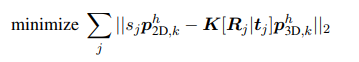# CVX problem processing in 3D reconstruction

As a student learning CVX problems for the first time, I need help when I meet a problem about 3D reconstruction.
Background:Extract K=11 points of J=12 images as data.
Known data:
11 points in the two-dimensional coordinate P_2D_k homogeneous (4 * 1 vector), camera matrix K (3 * 3 matrix), posture data [R_j | t_j] (4 * 4 matrix)